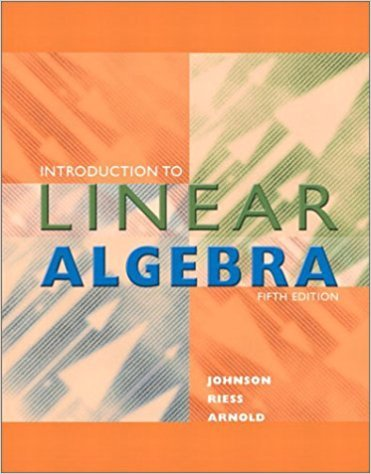×
Get Full Access to Introduction To Linear Algebra - 5 Edition - Chapter 1.4 - Problem 1e
Get Full Access to Introduction To Linear Algebra - 5 Edition - Chapter 1.4 - Problem 1e

×

# (a) set up the system of equations that describes trafficISBN: 9780201658590 59

## Solution for problem 1E Chapter 1.4

Introduction to Linear Algebra | 5th Edition

• Textbook Solutions
• 2901 Step-by-step solutions solved by professors and subject experts
• Get 24/7 help from StudySoup virtual teaching assistantsIntroduction to Linear Algebra | 5th Edition

4 5 1 284 Reviews
18
3
Problem 1E

(a) set up the system of equations that describes traffic flow; (b) determine the flows x1 , x2, and x3 if x4 = 100; and (c) determine the maximum and minimum values for x4 if all the flows are constrained to be nonnegative.

Step-by-Step Solution:
Step 1 of 3

a. rdrdicdn"fim 'lxt TL-l 'l

Step 2 of 3

Step 3 of 3

##### ISBN: 9780201658590

Unlock Textbook Solution